# WORKDAY Excel FunctionArticle byExcelMojo Team## What Is WORKDAY Function In Excel?

The WORKDAY Excel function helps users calculate the required date, keeping into account the number of working days before or after a given date, excluding the weekends and specified holidays. We can calculate invoice due dates and expected order delivery dates.

The WORKDAY Function In Excel is an inbuilt Date & Time function, which means we can insert the formula from the “Function Library” or enter it directly in the worksheet.

For example, the table below contains a set of dates and the number of days.

Select cell D2, enter the formula =WORKDAY(A2,B2), and press the “Enter” key. Then, drag the formula from cell D2 to D6.

In cell D5, edit the formula and insert excel cell reference C5 as the 3rd argument, i.e., holidays. So, the formula in D5 will then be updated to =WORKDAY(A5,B5,C5).

The output is shown above. Column E is for our reference that displays the formulas used in column D. The function excludes weekends (Saturdays and Sundays) while calculating the required dates.

However, row 5 highlights a holiday in cell C5, 4/7/2022. So, the WORKDAY Excel function calculation in the target cell D5 excludes the holiday with the weekends falling in the 15-day duration to return the date, 25/7/2022.

[Note: Set the output column’s format to Date. If it is General, we will get an incorrect output format.]

###### Key Takeaways
• The WORKDAY Excel function calculates a date, the number of working days before or after a given date.
• Ensure the data format of the target cell is Date. Otherwise, you will see the function output as a serial number equivalent to the resulting date value.
• We can use the WORKDAY function with other Excel functions, such as IF, TIME, and MOD.
• The function does not count the start_date as a working day. The WORKDAY Excel function returns a future date if this argument value is positive. And for a negative value of days, it returns a past date.

### WORKDAY() Excel Formula

The syntax of the WORKDAY Excel formula is:

The arguments of the WORKDAY Excel formula are:

• start_date: A date representing the starting date. It is a mandatory argument.
• days: The number of workdays, excluding weekends and specified holidays, before or after the start_date. It is a mandatory argument.
• holidays: A list of one or more holidays you require to exclude in the calculation. It can be an array of dates or an array constant of the serial numbers representing the dates. It is an optional argument.

### How To Use WORKDAY Excel Function?

We can use the WORKDAY Excel function in 2 methods, namely,

1. Access from the Excel ribbon.
2. Enter in the worksheet manually.

#### Method #1 – Access from the Excel ribbon

First, choose a cell for output → select the “Formulas” tab → go to the “Function Library” group → click the “Date & Time” drop-down → select the “WORKDAY” function, as shown below.

The “Function Arguments” window appears. Enter the Start_date, Days, and the Holidays arguments as values or cell references. Then, click “OK”, as shown below.

#### Method #2 – Enter in the worksheet manually

First, ensure all the date values in the source data are in the Date format.

1. Select the target cell for the output.
2. Type =WORKDAY( in the cell. [Alternatively, type =W and double-click the WORKDAY function from the Excel suggestions.]
3. Enter the values for the arguments.
4. Click “OK”.
5. Finally, press the “Enter” key.

We will understand the WORKDAY Excel function with a basic example for different datasets.

The below table shows two data sets, with all the column’s data format set to Date.

The steps to apply the WORKDAY Excel function are:

1. First, select cell B8, enter the formula =WORKDAY(B2,B3,B4:B5), and press the “Enter” key.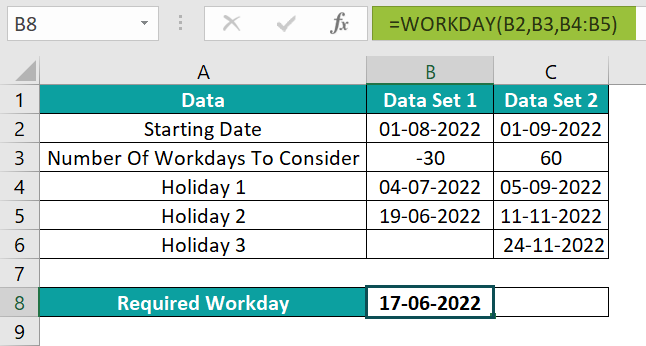[Alternatively, select cell B8 and click FormulasDate & TimeWORKDAY.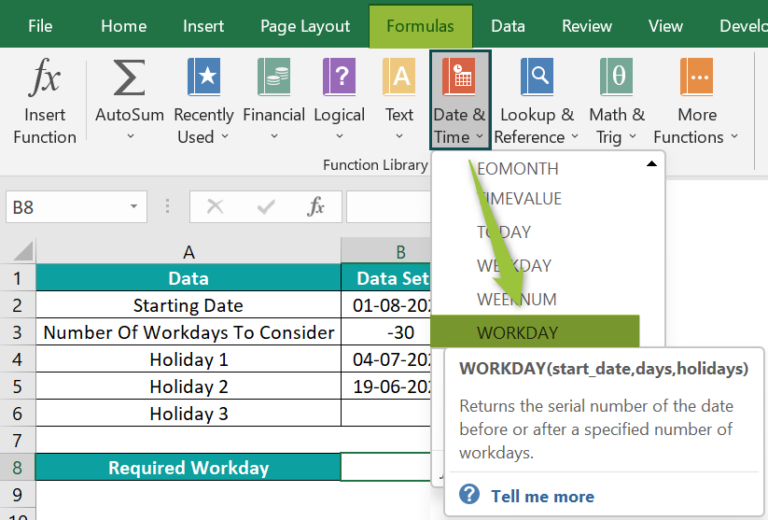Enter the three argument values in the Function Arguments window.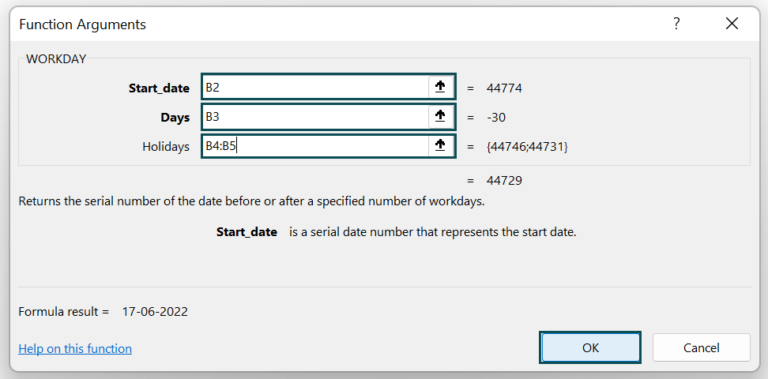And once you click OK, the function in the target cell B8 will get executed to give the result.]2. Select cell C8, enter the formula =WORKDAY(C2,C3,C4:C6), and press the “Enter” key.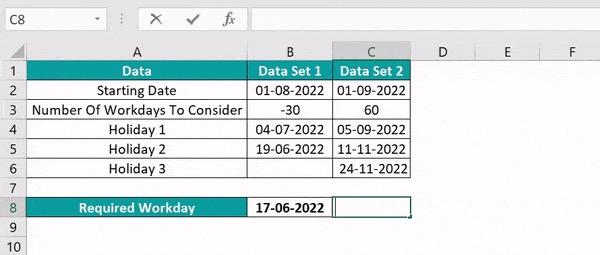We get the output as shown above.

[Output observation: The above formulas using the WORKDAY Excel function exclude weekends and holidays you specify in each dataset. And suppose a holiday falls on a weekend, then the function considers it a weekend, and the date is excluded while performing the calculations.

For instance, the holiday on 19/6/2022 falls on a Sunday. So, for Data Set 1, the WORKDAY() considers it a weekend date to return the date 30 days before 1/8/2022. And it ignores only one holiday date, 4/7/2022, along with the weekends.]

### Examples

We will understand advanced scenarios using WORKDAY Excel function.

#### Example #1

We will generate a sequence of working days using WORKDAY Excel function to determine the next six working days and display the corresponding day names.

The following table contains the first workday date in the Date format in Excel and the corresponding day name.

• Step 1: Select cell B2, enter the formula =A2, and press the “Enter” key. The output is shown below.
• Step 2: To format cell B2 to display the corresponding day’s name, select the “Home” tab → go to the “Number” group → click the “Number Format” drop-down → select the “More Number Formats…” option, as shown below.
• Step 3: In the “Format Cells” window that pops up, select the “Number” tab → click the “Custom” option on the left, in the “Category:” group → in the “Type:” group on the right, select the “dddd” option to display the date as a day àclick “OK”, as shown below.

We have now linked cell B2 with A2 and changed the date format of cell B2 to display the day’s name.

• Step 4: Now, select cell A3, enter the formula =WORKDAY(A2,1), and press the “Enter” key. The output is shown below.
• Step 5: Drag the formula from cell A3 to A8 using the fill handle.
• Step 6: Drag the formula and formatting from cell B2 to B8 using the fill handle.

The output is shown above. We can select both the cells, A2 and B2, and drag the formulas and the formatting simultaneously.

[Output Observation: In the above example, the source data does not highlight any holidays. So, we ignore the argument holidays in the WORKDAY functions used in the target cells A3:A8. And the day’s argument is 1 in all the formulas, as we need consecutive working days.

Now the output shows the workdays are consecutive, from 5/12/2022 to 9/12/2022. And, as the dates 10/12/2022 and 11/12/2022 fall on the weekend, WORKDAY() excludes them. So, instead, it returns the next two weekdays as the working days, 12/12/2022 and 13/12/2022, to complete the sequence.]

#### Example #2

We will use the WORKDAY and IF() excel function to determine whether a day is a workday or holiday.

The below table contains a list of dates and the holiday list.

The steps to apply the WORKDAY Excel function in the IF() function are as follows:

• Step 1: Select cell B2, enter the formula =IF(WORKDAY(A2-1,1,\$E\$3)=A2,”Working Day”,”Holiday”), and press the “Enter” key.
• Step 2: Drag the formula from cell B2 to B6 using the fill handle.

We get the output as shown above.

[Output Observation: Let us see how the above formula work in cell B6. First, in WORKDAY(), one gets deducted from 25/11/2022. Thus the WORKDAY() accepts 24/11/2022 as the input date. Then, considering the Holidays List, the function returns the next working date, 25/11/2022. And since the IF condition holds, the IF() returns Working Day as the output.

In the case of the target cell B5, one gets deducted from 24/11/2022 in the WORKDAY(). So, the WORKDAY() considers 23/11/2022 as start_date and checks the Holidays List to return the next working day as 25/11/2022. The reason is that WORKDAY() ignores the holiday, 24/11/2022. And as the IF condition is FALSE, the function output is Holiday.]

#### Example #3

We will use the WORKDAY Excel function with IF, TIME, HOUR, MINUTE, SECOND, and MOD functions.

The table below includes a starting date and time, the number of working hours you have to add, workings hours start and end time, and the holidays you must exclude.

The steps to apply the WORKDAY Excel function with hours included in the output are:

• Step 1: Choose cell C3 → select the “Home” tab → go to the “Number” group → click the “Number Format” drop-down à select the “More Number Formats…” option, as shown below.
• Step 2: In the “Format Cells” window that pops up, select the “Number” tab → click the “Custom” option on the left, in the “Category:” group → in the “Type:” group on the right, select the “m/d/yyyy h:mm” option → click “OK”, as shown below.
• Step 3: Select cell C3, enter the formula

=WORKDAY(A3,INT(B3/9)+IF(TIME(HOUR(A3),MINUTE(A3),SECOND(A3))+TIME(MOD (B3,9),MOD(MOD(B3,9),1)*60,0)>\$F\$3,1,0),\$G\$3:\$G\$3)+IF(TIME(HOUR(A3),MINUTE(A 3),SECOND(A3))+TIME(MOD(B3,9),MOD(MOD(B3,9),1)*60,0)>\$F\$3,\$E\$3+TIME(HOUR( A3),MINUTE(A3),SECOND(A3))+TIME(MOD(B3,9),MOD(MOD(B3,9),1)*60,0)-\$F\$3,TIME (HOUR(A3),MINUTE(A3),SECOND(A3))+TIME(MOD(B3,9),MOD(MOD(B3,9),1)*60,0)), and press the “Enter” key.

We get the output as shown above.

[Output Observation: In the above example, the start date is 1/7/2022, a Friday. So, considering the holiday, 4/7/2022, the WORKDAY() ignores the weekend and the specified holiday to return to the next working date or end date, 5/7/2022. On the other hand, the remaining formula adds the required business hours to the start time and determines the end time.]

### Important Things to Note

• The WORKDAY Excel function excludes the weekends and holidays you specify while calculating the working date. Also, it does not count the start_date as a work day.
• Avoid entering the dates as text values. Instead, use the DATE() to enter them into the source data cells. And if you enter a non-integer value for the argument days, it will get shortened.
• For invalid start_date or holidays arguments or a non-numeric days argument, the WORKDAY() will return the #VALUE! error.
• Suppose the WORKDAY() determines an invalid date, we will get the #NUM! error.

1. Where is WORKDAY in Excel?

WORKDAY in Excel is in the Formulas tab. Click FormulasDate & Time WORKDAY.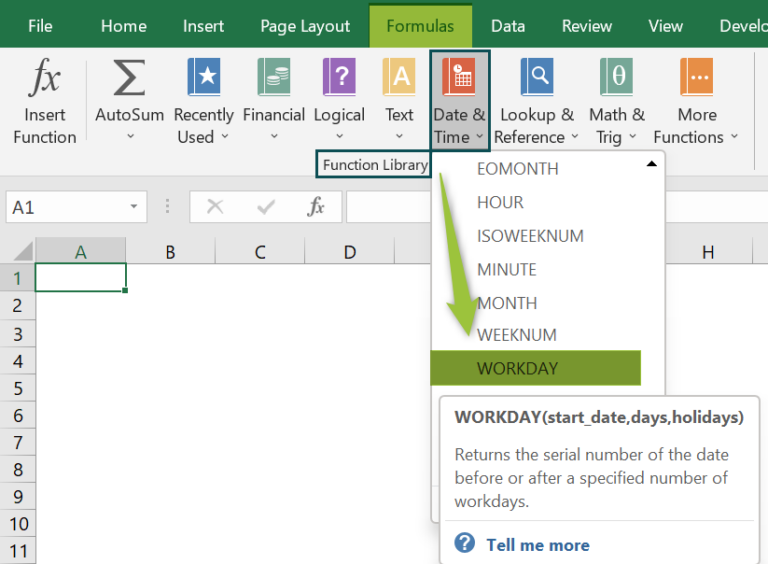2. Does the Excel WORKDAY function include holidays?

The Excel WORKDAY function includes the holidays while determining the work day if you do not specify the holidays list explicitly.

The WORKDAY() argument, holidays, is optional. And if you provide the cell range containing holidays to the function as an argument, the function excludes them from the workday calculations. Otherwise, it includes holidays in the evaluations.

3. Why does the WORKDAY Excel function not work?

The WORKDAY Excel function does not work due to the following reasons:
· If the argument start_date or holidays is invalid.
· When the argument, days, is non-numeric.
· The WORKDAY() determined date is invalid.

4. How to use the WORKDAY() in Excel VBA?

We can use the WORKDAY() in Excel with VBA to determine the corresponding work days.

In the following table, we have a list of dates, the number of days, and the holidays to exclude from the working day calculations.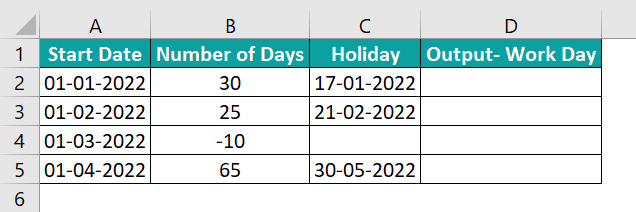The steps to use the WORKDAY() in VBA are:

1: With the worksheet with the above table, press the keys Alt+F11 to open the VBA Editor.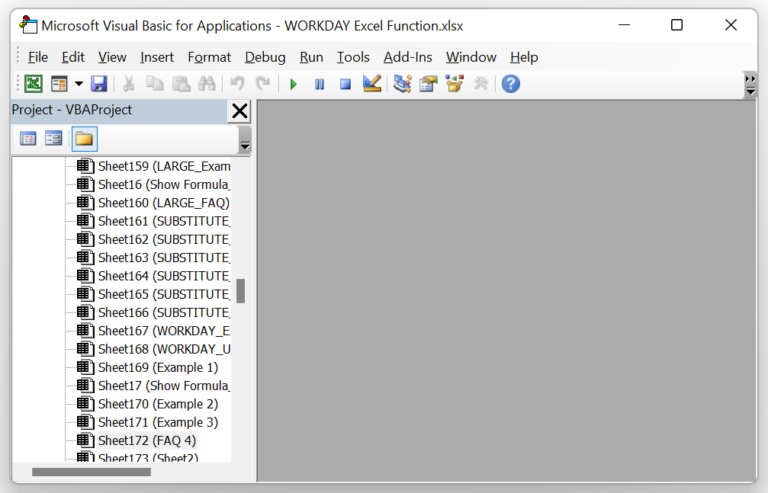2: Then, choose the required VBAProject from the left menu and click InsertModule to open the Module1 window, where you need to enter the VBA code.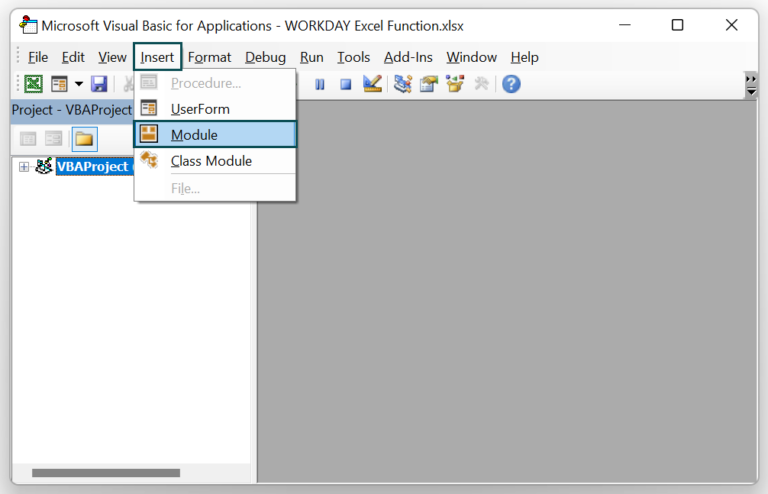The Module window opens as shown below.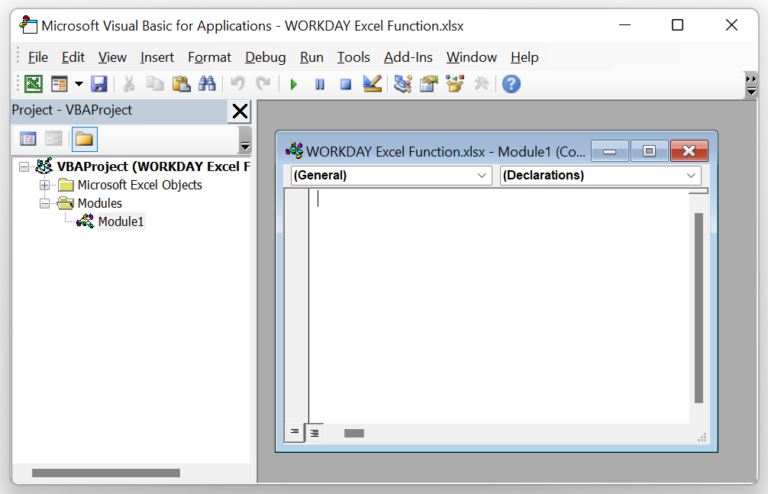3: Enter the VBA code, shown in the image below, in the Module1 window.

Sub Workday_fn()
Dim wd As Worksheet
Set wd = Worksheets(“FAQ 4”)
wd.Range(“D2”) = Application.WorksheetFunction.WorkDay(wd.Range(“A2”), wd.Range(“B3”), wd.Range(“C2”))
wd.Range(“D3”) = Application.WorksheetFunction.WorkDay(wd.Range(“A3”), wd.Range(“B3”), wd.Range(“C3”))
wd.Range(“D4”) = Application.WorksheetFunction.WorkDay(wd.Range(“A4”), wd.Range(“B4”))
wd.Range(“D5”) = Application.WorksheetFunction.WorkDay(wd.Range(“A5”), wd.Range(“B5”), wd.Range(“C5”))
End Sub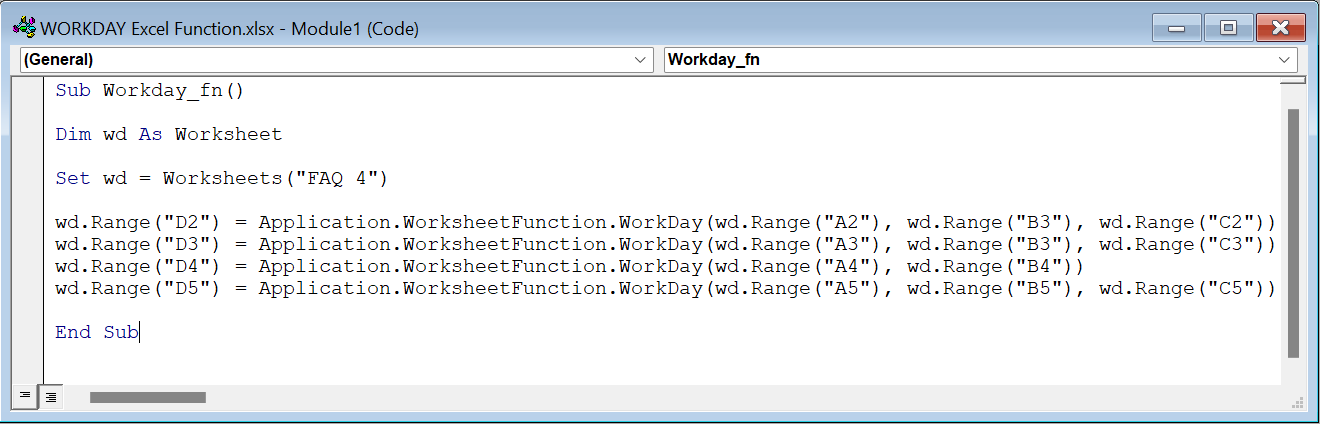4: Click the Run Sub/UserForm icon in the menu to run the commands.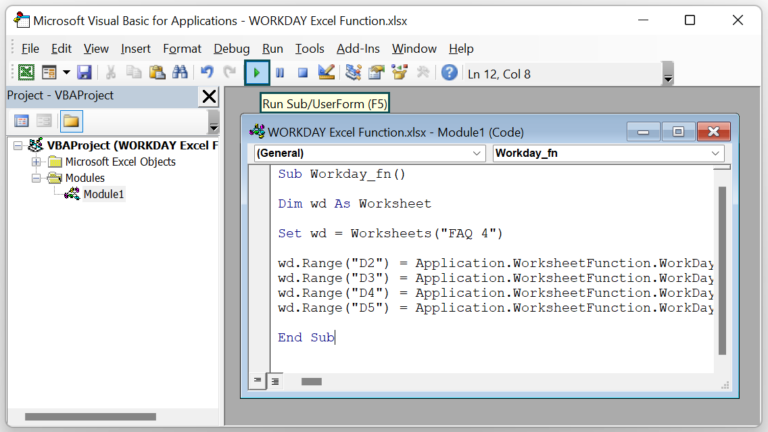In the worksheet, we will get the output in the target cells as shown below: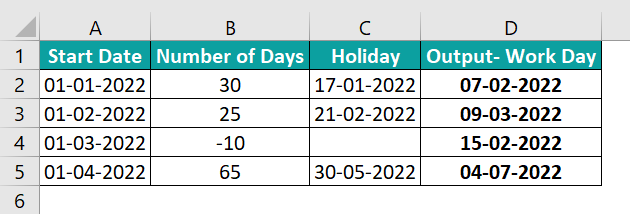[Output Observation: In the target cells, the WORKDAY() excludes the weekends and the specified holidays while returning the respective future work days as the output.

But in the case of row 4, you need a past work day from1/3/2022. And 21/2/2022 is a holiday that falls in the given number of days range. But as per the requirement, the WORKDAY() ignores the optional argument holidays. Thus, the function counts ten working days in the past from 1/3/2022, excluding only the weekends to return the work day 15/2/2022.]